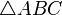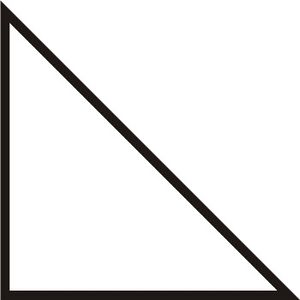# Triangle facts for kids

Kids Encyclopedia Facts

A triangle is a shape, or a part of two dimensional space. It has three straight sides and three vertices. The three angles of a triangle always add up to 180° (180 degrees). It is the polygon with the least possible number of sides. A triangle with vertices A, B, C is written as$\triangle ABC$.

## Types of trianglesThis is an isosceles right triangle, because it has a right angle and two of its sides have the same length.

Triangles can be grouped according to how many of their sides are equal:

• if all the three sides of a triangle have the same length, then it is an equilateral triangle.
• if a triangle has two sides with the same length, then it is an isosceles triangle.
• if all the three sides of a triangle have different lengths, then we have a scalene triangle.

Triangles can also be grouped by their angles:

• if a triangle has a right angle, that is, if one of the angles of that triangle measures 90° (90 degrees), then it is a right triangle. The opposite side to the right angle is the hypotenuse.
• if a triangle has an obtuse angle, that is, if one of the angles of that triangle is larger than 90°, then it is an obtuse triangle.
• if a triangle has only acute angles, that is, if all the angles of that triangle are less than 90°, then it is an acute triangle.

## Uses

Triangles are extremely useful. The study of geometry related to triangles is called trigonometry. Some people have spent their entire lives studying triangles for use in trigonometry.

Modern computers usually use triangles to make more complex graphic images or shapes.

## Images for kidsTriangle Facts for Kids. Kiddle Encyclopedia.